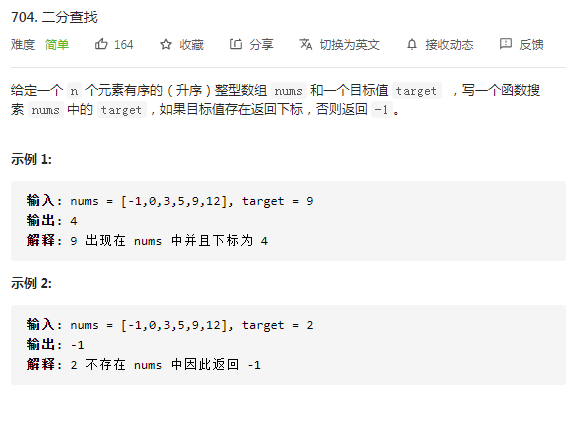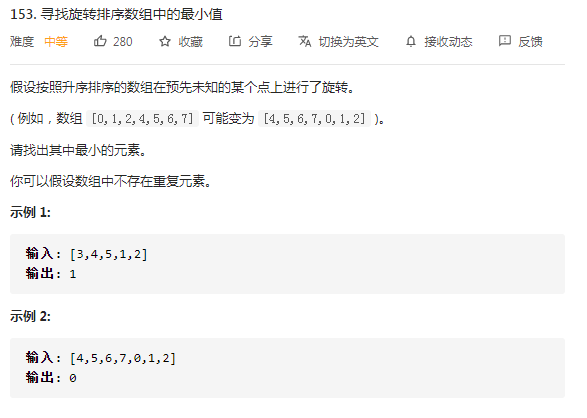# vcjmhg 的个人博客

## 二分查找及其应用# 框架与说明

## 通用二分查找框架

``````11. 初始化：为left和right赋值
22. 循环退出条件
33. 比较中值和目标值关系，分情况处理
4	1. 相等
5	2. 小于
6	3. 大于
``````

`````` 1int binarySearch(int [] nums,int target) {
2    int left = 0, right = ...;
3
4    while(...){
5        //防止溢出等同于mid = (left +right)/2
6        int mid = left + (right - left)/2;
7        if(nums[mid] == target) {
8            ...
9        }else if (nums[mid] < target) {
10            left = ...
11        }else if (nums[mid] > target) {
12            right = ...
13        }
14    }
15    return ...
16}
``````

## 基本二分查找`````` 1public int binarySearch(int[] nums, int target) {
2    // 初始化，细节一：right此处复制为nums.length - 1，
3    //相当于搜索区间为[left,righty]
4    int left = 0, right = nums.length - 1;
5    // 循环退出条件，细节二：由于细节一的原因，此处要使用left<=right
6    //，从而保证能够搜索到right的位置
7    while (left <= right) {
8      // 计算中值
9      int mid = left + (right - left) / 2;
10      // 查找到目标结果直接返回对应索引的位置
11      if (nums[mid] == target) {
12        return mid;
13      } else if (nums[mid] < target) {
14        // 细节三：因为已经验证过mid位置，因此需要从[mid+1，right]区间开始查找
15        left = mid + 1;
16      } else if (nums[mid] > target) {
17        // 细节四：原因同细节三
18        right = mid - 1;
19      }
20    }
21    // 区间内所有值都已搜索完毕，直接返回-1
22    return -1;
23  }
``````

1. 为什么 while 循环的条件中是 <=，而不是 < ?

1. 为什么 left = mid + 1,right = mid - 1?我看有的代码是 right = mid 或者 left = mid，没有这些加加减减，到底怎么回事，怎么判断？

## 左边界查找

`````` 1public int leftBound(int[] nums, int target) {
2    // 细节1：right赋值为length，意味着搜索区间范围左闭右开
3    int left = 0, right = nums.length;
4    // 细节2
5    while (left < right) {
6        int mid = left + (right - left) / 2;
7        if (nums[mid] == target) {
8            // 细节3
9            right = mid;
10        } else if (nums[mid] < target) {
11            left = mid + 1;
12        } else if (nums[mid] > target) {
13            // 细节4
14            right = mid;
15        }
16    }
17    // 细节5
18    return nums[left] == target ? left : -1;
19}
``````

1. 为什么 while(left < right) 而不是 <= ?

1. 为何返回 left？

## 右边界查找

`````` 1public int rightBound(int[] nums, int target) {
2    int left = 0, right = nums.length;
3    while (left < right) {
4        int mid = left + (right - left) / 2;
5        if (nums[mid] == target) {
6            //细节1
7            left = mid + 1;
8        } else if (nums[mid] < target) {
9            left = mid + 1;
10        } else if (nums[mid] > target) {
11            right = mid;
12        }
13    }
14    //细节2
15    return nums[left - 1] == target ? left - 1 : -1;
16}
``````

1. 中点值和目标值相同时，不直接返回而是要通过 `left = mid + 1` 将查找区间向右逼近，进而才能查找最右侧的值
2. 返回值是 `left -1` ，主要是因为，在找到目标值之后做了一个 left = mid +1 操作，从而实际我们想要的 mid 的值为 left -1。

# 典型例题

## 1. 寻找旋转排序数组中的最小值`````` 1 public int findMin(int[] nums) {
2    int left = 0, right = nums.length - 1;
3    int min = nums[left];
4    while (left <= right) {
5      int mid = ((right - left) >> 1) + left;
6      if (nums[mid] < min) {
7        min = nums[mid];
8      }
9      if (nums[left] < nums[right]) {
10        right = mid - 1;
11      } else if (nums[left] > nums[right]) {
12        // 证明最小值在mid的前面
13        if (mid > left && nums[mid] > nums[mid - 1]) {
14          right = mid - 1;
15        } else {
16          left = mid + 1;
17        }
18      } else if (nums[left] == nums[right]) {
19        return min;
20      }
21    }
22    return min;
``````